# Two workers

Two workers make 138 parts/items. The first one produces 30% more than the second. How many items will each produce?

Correct result:

a =  78
b =  60

#### Solution:

a+b=138
a = b + 30/100b

a+b=138
a = b + 30/100•b

a+b = 138
100a-130b = 0

a = 78
b = 60

Our linear equations calculator calculates it.We would be pleased if you find an error in the word problem, spelling mistakes, or inaccuracies and send it to us. Thank you!Tips to related online calculators
Do you have a linear equation or system of equations and looking for its solution? Or do you have quadratic equation?

## Next similar math problems:

• 3 days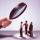Worker checked 2,950 products in 3 days. Second day checked 25% more than the first day. The third day 15% more products than the second day. How many products he checked in each day.
• Three piles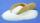100 kg of sugar we divide into three piles. The first pile is small. If we added to second 2 kg of sugar it would have 25% more sugar than the first pile. If we added to third pile 3 kg of sugar it would have 20% more sugar than the 2nd pile. How many kil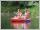Students of canoeists trip traveled in three days 102 km. Second day traveled 15% more than first day and at the third day 9 km over the second day. How many kilometers traveled each day?
• Part-time workersThree part-time workers received CZK 1,235 for their work. The first received 20% less than the second and the third received 45 CZK more than the second. Determine how many crowns (CZK) each of them received.
• EmployeesThere are 1116 people working in three factory halls. In the first one, there are 18% more than the third, and 60 persons more than the second. How many employees work in individual halls?
• Three workersThree workers were rewarded CZK 9200 and the money divided by the work they have done. First worker to get twice than the second, the second three times more than the third. How much money each worker received?
• RewardThree workers have shared a common reward 13110 CZK follows: first worker got 35% less than the second and third worker got 20% more than the second worker. How much got each worker?
• Three workers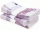The three workers received € 2,850 together for the work done. They divided them according to the time worked so that the first received 20% less than the second and the third € 50 more than the second. How much EUR did each worker receive?
• The farmer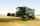The farmer harvested 840 tons of grain in 2006, which was 44% less than in 2005, and one-fifth more than in 2004. How many tons of grain harvested in years 2005 and 2004?
• Tickets1260 tickets sold. On the first day, 80% was sold on the second day was sold. How many tickets were sold first and how much the next day?
• Two math problems1) The sum of twice a number and -6 is nine more than the opposite of that number. Find the number. 2) A collection of 27 coins, all nickels, and dimes, is worth \$2.10. How many of each coin are there? The dime, in United States usage, is a ten-cent coin.
• Apartments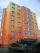Apartment on the first floor was 10% more expensive than the same apartment on the second floor. The difference was 105 Kc annually. Calculate the annual rent of the apartment in the first floor and from apartment on the second floor.
• RemunerationTotal remuneration for workers is 47 000 CZK. The second worker received 20% more than the first and third 4000 CZK less than a second employee. How many CZK each received?
• A man 2A man divides \$10,000 into two investments, one at 10% and the other at 30%. Find how much is invested at each rate so that the two investments produce the same income annually.
• Container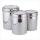First day got off 1/4 water from full container, second day 1/5 of the remaining water. How many percent remain container filled?
• ClassroomThere are eighty more girls in the class than boys. Boys are 40 percent and girls are 60 percent. How many are boys and how many girls?
• Scouts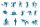Three scouts went on a three day trip. The second day went 4 km more than the first day. The third day went two times less than the first day. They went along 54 km. How many kilometers went every day together?